• 本资源为运用盛金公式求解一元三次方程，网上我找了半天，竟然没有一个代码完全求解或者精度达到要求，本资源亲证可求解并可达到刁钻的精度要求，包括两个word文件，用来讲解公式，另外一个为.cpp文件实现求解过程~
• ## 盛金公式入门

千次阅读 2021-05-01 07:30:08
盛金公式 昨天做一道二分的题目，就是一元三次方程求三个根，然后从小到大输出。 我发现好多大佬都是用的盛金公式或者牛顿迭代(听说比二分快)。 所以我打算学习一下，对于盛金公式我只找了百度和作业帮的资料，还有...

盛金公式
昨天做一道二分的题目，就是一元三次方程求三个根，然后从小到大输出。
我发现好多大佬都是用的盛金公式或者牛顿迭代(听说比二分快)。
所以我打算学习一下，对于盛金公式我只找了百度和作业帮的资料，还有一些学术网站的证明(肯定不会看%%%)
所以我打算简单的介绍一下盛金公式。
假设一元三次方程：aX3+bX2+cX+d=0
首先和一元二次方程类似，他也有重根判别式：A=b*b-3*a*c;
B=b*c-9*a*d;
C=c*c-3*b*d;
derta=B2-4*A*C;//总判别式
当A=B=0时：
盛金公式1：X1=X2=X3=-b/(3*a)=-c/b=-3d/c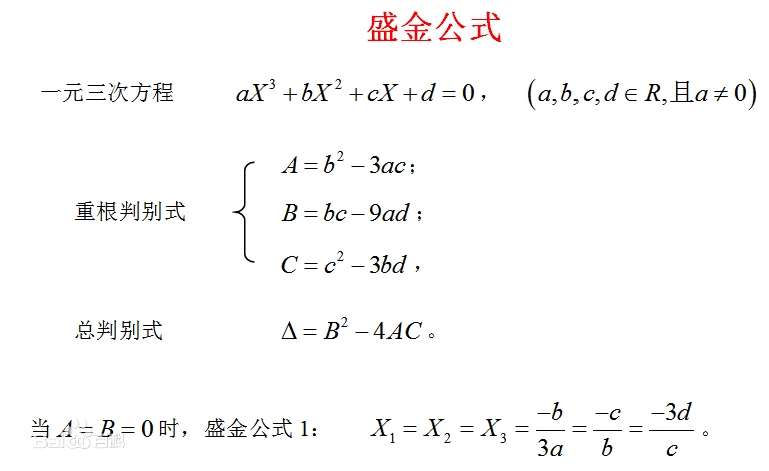当derta>0时：
盛金公式2：Y1,2=A*b+3*a*(-B±sqrt(B2-4*AC))/2;   i2=1
X1=(-b-(pow(Y1,1/3)+pow(Y2),1/3))/(3*a);
X2,3=(-b+(pow(Y1,1/3)+pow(Y2,1/3))±sqrt(3)*(pow(Y1,1/3)-pow(Y2,1/3)*i/2)/2)/(3*a);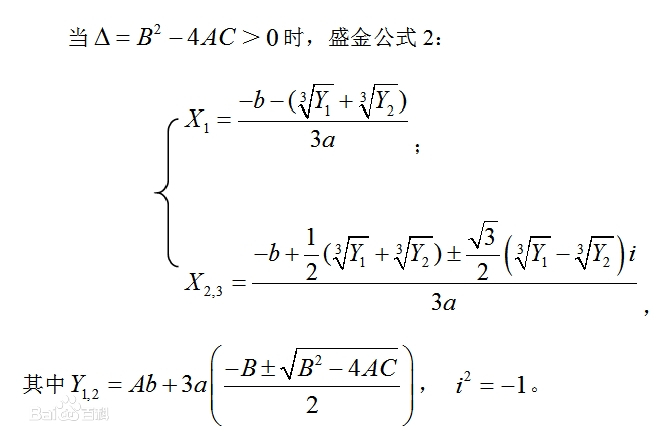当derta=0时：
盛金公式3：K=B/A;(A!=0)
X1=-b/a+K;
X2=X3=-K/2;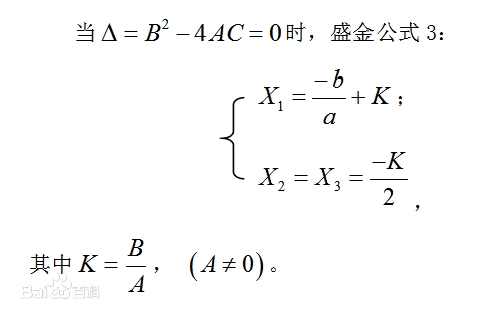当derta<0时：
盛金公式4：T=(2*A*b-3*a*B)/(2*sqrt(A*A*A))　　θ=arccos(T)//C语言中为acos(T)  (A>0&&-1<T<1)
X1=(-b-2*sqrt(A)*cos(θ/3))/(3*a);
X2,3=(-b+sqrt(A)*(cos(θ/3)±sqrt(3)sin(θ/3)))/(3*a);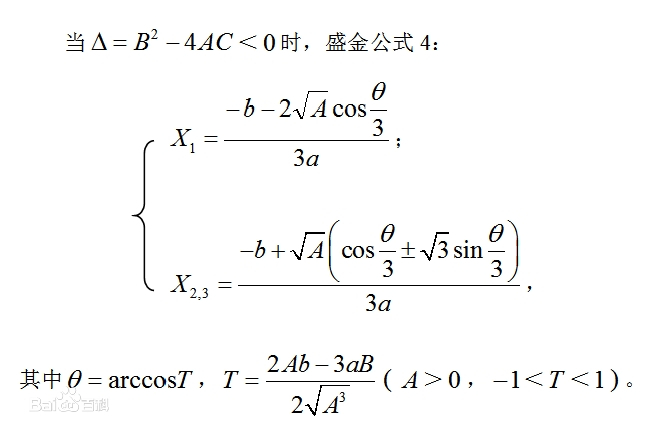然后我们就能根据不同的要求求出一元三次的三个解。
是不是觉得盛金公式法有点像一元二次方程的万能公式法。
具体的练习：https://www.luogu.com.cn/problem/P1024    //就是一道经典的盛金公式求解题目(但是好像暴力能过，因为精度很小)
题解：https://www.cnblogs.com/Mangata/p/13520980.html
但是盛金公式求解有个问题(在计算机上)，因为盛金公式可能会用到sin,cos等三角函数，就会很容易造成精度不足，所以请谨慎使用
能二分尽量二分(毕竟精度能自己控制=_=)。

展开全文python 算法 机器学习 http
• 一元三次方程求解(求根) - 盛金公式法 一、引言 只含有一个未知数（即“元”），并且未知数的最高次数为3（即“次”）的整式方程叫做一元三次方程（英文名：cubic equation in one unknown）。一元三次方程的...
                    一元三次方程求解(求根) - 盛金公式法

一、引言
只含有一个未知数（即“元”），并且未知数的最高次数为3（即“次”）的整式方程叫做一元三次方程（英文名：cubic equation in one unknown）。一元三次方程的标准形式（即所有一元三次方程经整理都能得到的形式）是ax3+bx2+cx+d=0（a，b，c，d为常数，x为未知数，且a≠0）。一元三次方程的公式解法有卡尔丹公式法。(--引自百度百科)；
由于卡尔丹公式法和多项式求解的方法不容易理解，不容易用程序实现，经过文献的查找了解到盛金公式求解一元三次方程，经验证求根正确可以引用。

二、盛金公式简介
80年代，中国中学数学教师范盛金对解一元三次方程问题进行了深入的研究和探索，发明了比卡尔丹公式更适用的新根公式 - 盛金公式，并建立了简明的、直观的、使用的新判别法 - 盛金判别法，同时提出了盛金定理；盛金定理清晰地回答了解一元三次方程的疑惑问题，并且很有趣味。
盛金公式的特点是由最简重根判别式：, , ；和总判别式来构成，体现了数学的有序、对称、和谐与简洁美。

三、盛金公式表达式
一元三次方程式为：
(1)
重根判别式为：
(2)
总判别式为：
(3)
盛金判别法的结论为：
1.  条件一： 当A = B = 0时，方程有一个三重实根
(4)

2.  条件二： 当 时 ，方程有一个实根和一个共轭虚根
(5)
其中:
;

3.  条件三：当时，方程有三个实根，其中有一个两重根
(6)
其中：
;

4.  条件四： 当时，方程有三个不相等的实根
(7)
其中：

四、盛金公式求解程序流程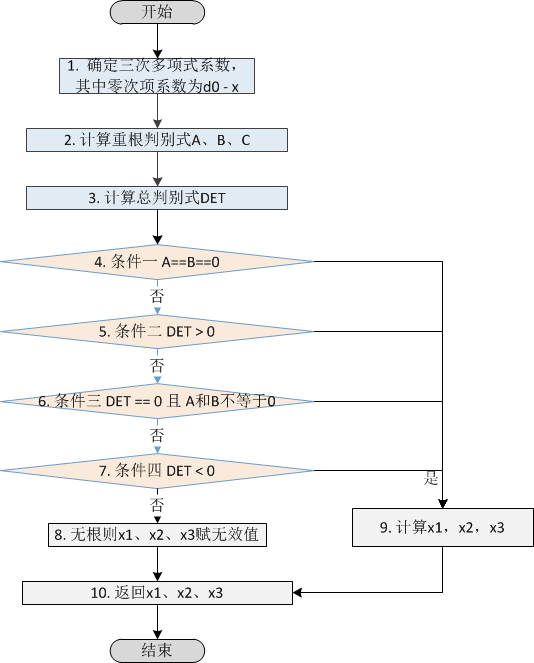五、java和matlab实例代码

matlab代码
function x = solve3Polynomial(a, b, c, d)
%%%%%%%%%%%%%%%%%%%%%%%%%%%%%%%%%%%%%%%%%%%%%%%%%%%%%%%%%%%%%%%%%%%%%%%%%%%
%
%  funtion x = solve3Polynomial(a, b, c, d)
%
%> @brief 利用盛金公式求解三阶多项式的解.
%>
%> @details 输入三阶多项式系数，求解ax^3 + bx^2 + cx^1 + d = 0的根
%>          参考文献：范盛金. 一元三次方程的新求根公式与新判别法[J]. 海南师范学院学报,1989,2(2):91-98.
%>
%> @param[out]   x      求解完成的三个根x1，x2，x3
%> @param[in]    a      三次项系数
%> @param[in]    b      二次项系数
%> @param[in]    c      一次项系数
%> @param[in]    d      零次项系数
%
%%%%%%%%%%%%%%%%%%%%%%%%%%%%%%%%%%%%%%%%%%%%%%%%%%%%%%%%%%%%%%%%%%%%%%%%%%%

%% 重根判别式
A = b*b - 3*a*c;   if abs(A) < 1e-14;    A = 0;  end
B = b*c - 9*a*d;   if abs(B) < 1e-14;    B = 0;  end
C = c*c - 3*b*d;   if abs(C) < 1e-14;    C = 0;  end

%% 总判别式
DET = B*B - 4*A*C; if abs(DET) < 1e-14;  DET = 0;  end

%% 条件一
if (A == 0) && (B == 0)
x1 = -c/b;      x2 = x1 ;    x3 = x1;
end

%% 条件二
if DET > 0
Y1 = A*b + 1.5*a*(-B + sqrt(DET));
Y2 = A*b + 1.5*a*(-B - sqrt(DET));
y1 = nthroot(Y1,3);  y2 = nthroot(Y2,3);
x1 = (-b-y1-y2)/(3*a);
vec1 = (-b + 0.5*(y1 + y2))/(3*a);
vec2 = 0.5*sqrt(3)*(y1 - y2)/(3*a);
x2 = complex(vec1, vec2);
x3 = complex(vec1, -vec2);
clear Y1 Y2 y1 y2 vec1 vec2;
end
%% 条件三
if DET == 0 && (A ~= 0) && (B ~= 0)
K = (b*c-9*a*d)/(b*b - 3*a*c); K = round(K,14);
x1 = -b/a + K;   x2 = -0.5*K;   x3 = x2;
end

%% 条件四
if DET < 0
sqA = sqrt(A);
T = (A*b - 1.5*a*B)/(A*sqA);
theta = acos(T);
csth  = cos(theta/3);
sn3th = sqrt(3)*sin(theta/3);
x1 = (-b - 2*sqA*csth)/(3*a);
x2 = (-b + sqA*(csth + sn3th))/(3*a);
x3 = (-b + sqA*(csth - sn3th))/(3*a);
clear sqA T theta csth sn3th;
end
x = [x1;  x2;  x3];
end

java代码
 public double solve3Polynomial(double a, double b, double c, double d)
{
double x1 = 0.0, x2 = 0.0, x3 = 0.0;
double A = 0.0, B = 0.0 , C =0.0 , DET = 0.0;

// 1. 计算重根判别式
A = b*b - 3*a*c;
if(Math.abs(A) < 1e-14)
{
A = 0.0;
}

B = b*c - 9*a*d;
if(Math.abs(B) < 1e-14)
{
B = 0.0;
}

C = c*c - 3*b*d;
if(Math.abs(C) < 1e-14)
{
C = 0.0;
}

// 2. 计算总判别式
DET = B*B - 4*A*C;
if(Math.abs(DET) < 1e-14)
{
DET = 0;
}

// 3. 条件一，计算根
if((A == 0) && (B == 0))
{
x1 = (-1*c)/b;
x2 = x1;
x3 = x1;
//Log.i("roots", "条件一:" + x1 );
}

// 4. 条件二，计算根
if(DET > 0)
{
double Y1 = A*b + 1.5*a*(-1*B + Math.sqrt(DET));
double Y2 = A*b + 1.5*a*(-1*B - Math.sqrt(DET));
//Log.i("SQSddd", "Y1: " + Y1 + " Y2: " + Y2);
double y1 = getCubeRoot(Y1);
double y2 = getCubeRoot(Y2);
x1 = (-1.0*b-(y1+y2))/(3.0*a);				// 一个实根
double vec1 = (-1*b + 0.5*(y1 + y2))/(3*a);
double vec2 = 0.5*Math.sqrt(y1 - y2)/(3*a);
//    		x2 = Math.
double x3_real = 0.0, x2_real = 0.0;		// 实部
x2_real = (-b + getCubeRoot(Y1)) / (3 * a);
x3_real = x2_real;
double x2_virtual = 0.0, x3_virtual = 0.0;  // 虚部
x2_virtual = ((Math.sqrt(3) / 2) * (y1 - y2 )) / (3 * a);
x3_virtual = -x2_virtual;
//Log.i("roots", "条件二:" + x1 );
}

// 5. 条件三，计算根
if(DET == 0 && A != 0 && B != 0)
{
double K = (b*c - 9*a*d)/(b*b -3*a*c);
K = Math.round(K);
x1 = (-1.0*b)/a + K;
x2 = -1*0.5*K;
x3 = x2;
//Log.i("roots", "条件三:" + x1 );
}

// 6.条件四，计算根
if(DET < 0)
{
double sqA = Math.sqrt(A);
double T = (A*b - 1.5*a*B)/(A*sqA);
double theta = Math.acos(T);
double csth = Math.cos(theta/3);
double sn3th = Math.sqrt(3)*Math.sin(theta/3);
x1 = (-1*b - 2*sqA*csth)/(3*a);
x2 = (-1*b + sqA*(csth + sn3th))/(3*a);
x3 = (-1*b + sqA*(csth - sn3th))/(3*a);
//Log.i("SQSd", "条件四:" + x1 );
}

// 7. 返回计算结果
return x1;
}

public double getCubeRoot(double value) {
if (value < 0) {
return -Math.pow(-value, 1.0/3.0);
} else if (value == 0) {
return 0;
} else {
return Math.pow(value, 1.0/3.0);
}

}


展开全文一元三次方程 一元三次方程求解
• C++\一元三次方程的盛金公式解题法讲得很详细，比喻生动恰当，逻辑性强，透彻，清晰，易懂
•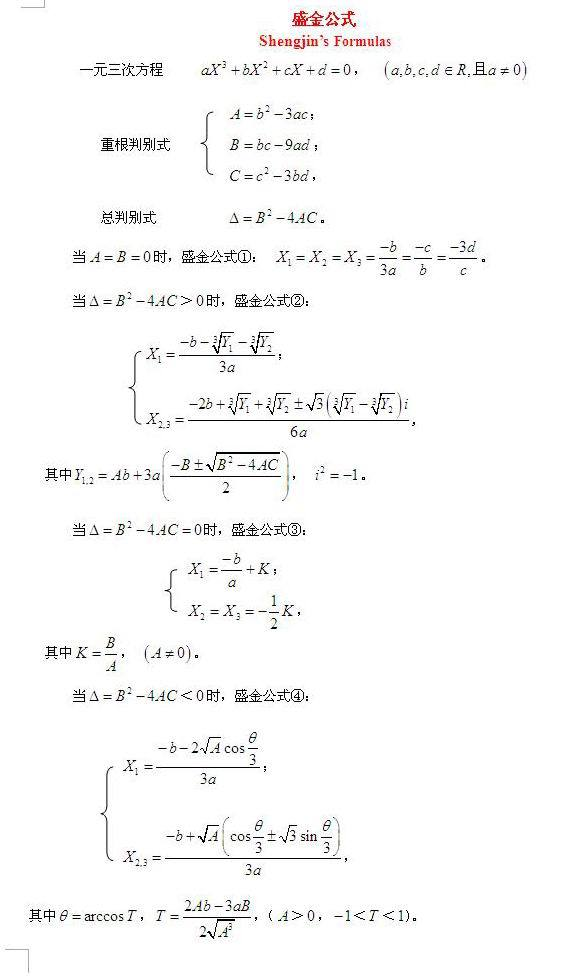展开全文数学
• //当A=B=0时，公式1.+定理6 if ((A==0&&B==0)||(delt==0&&A==0)) { X1 = -b/(3*a); X2 = X1; X3 = X1; } //Δ=B2－4AC>0时，公式2 if (delt>0) { double Y1 = A*b+3*a*((-B+pow(delt,0.5))/2); ...
原理参考-百度百科（http://baike.baidu.com/link?url=eA-bEvbcOBM2XmA4rzIG-lgci4MQdQcr7lCzCHBW-qG-qcPaDNovXp_jYxS2FUjlrOh1obH_D3Yv6ME2JYOxPyCgKhHIaXCHe-k9e69_c2tUxMTY90Z5-Oh9pZ-8LRf_）
先说一下中间出现的问题，开三次方的时候不能直接pow(x,1.0/3.0); 要分x大于零小于零，pow只接受大于零的参数。
另外，由于工程中只考虑实数，这里复数没有实现。
//cubicSolute.h


#pragma once
class cubicSolute
{
public:
cubicSolute(void);
~cubicSolute(void);
void setCoefficient(double a,double b,double c,double d)//设方程系数
{
this->a =a;
this->b =b;
this->c =c;
this->d =d;
}
void judge();
double getX1(){return X1;}
double getX2(){return X2;}
double getX3(){return X3;}

private:
double a,b,c,d;
double A,B,C;
double delt;//判别式
double X1,X2,X3;
};//cubicSolute.cpp

#include "cubicSolute.h"
#include <math.h>

cubicSolute::cubicSolute(void)
{
}

cubicSolute::~cubicSolute(void)
{
}

//Δ根的判别式
void cubicSolute::judge()
{
A = pow(b,2)-3*a*c;
B = b*c-9*a*d;
C = pow(c,2)-3*b*d;
delt = pow(B,2)-4*A*C;

//当A=B=0时，公式1.+定理6
if ((A==0&&B==0)||(delt==0&&A==0))
{
X1 = -b/(3*a);
X2 = X1;
X3 = X1;
}

//Δ=B2－4AC>0时，公式2
if (delt>0)
{
double Y1 = A*b+3*a*((-B+pow(delt,0.5))/2);
double Y2 = A*b+3*a*((-B-pow(delt,0.5))/2);
//解决负数开三方出问题
double Y1_three,Y2_three;
if (Y1<0)
Y1_three = - pow(-Y1,1.0/3.0);
else
Y1_three = pow(Y1,1.0/3.0);
if (Y2<0)
Y2_three = - pow(-Y2,1.0/3.0);
else
Y2_three = pow(Y2,1.0/3.0);
X1 = (-b-(Y1_three+Y2_three))/(3*a);
//X1,X2为复数，这里不需要，未实现。
}

//当Δ=B2－4AC=0时，公式3
if (delt==0&&(A!=0))
{
double K = B/A;
X1 = -b/a + K;
X2 = -K/2.0;
X3 = X2;
}

//当Δ=B2－4AC<0时，公式4
if (delt<0)
{
double T = (2*A*b-3*a*B)/(2*A*pow(A,0.5));//（A>0，－1<T<1）
double theita = acos(T);
X1 = (-b-2*pow(A,0.5)*cos(theita/3.0))/(3*a);
X2 = (-b+pow(A,0.5)*(cos(theita/3.0)+pow(3,0.5)*sin(theita/3.0)))/(3*a);
X3 = (-b+pow(A,0.5)*(cos(theita/3.0)-pow(3,0.5)*sin(theita/3.0)))/(3*a);
}

}

测试ok


展开全文一元三次方程
• class dd2 { private static readonly double dsr3 = Math.... /// 盛金公式求解一元三次方程,ax^3+bx^2+cx+d=0。 /// </summary> /// <param name="a">三次系数</param> /// <param na...c#
• MATLAB实现一元三次方程求解/盛金公式 一元三次方程求解中，1945年卡尔丹诺把冯塔纳的三次方程求根公式发表出来，但该公式形式比较复杂，直观性也较差。1989年范盛金对一元三次方程求解进行了深入的研究和探索，提出...一元三次方程 MATLAB代码
• ## 盛金公式

千次阅读 2009-07-25 22:42:00
盛金公式最近学习了几篇编程解线代方面的文章,为解多次方程无意在网上发现了一个据称可解所有实系数三次方程的"盛金公式",转贴如下:一元三次方程aX3＋bX2＋cX＋d=0，（a，b，c，d∈R，且a≠0）。　重根判别式：　A=...编程 c
• /// /// 盛金公式求解一元三次方程 /// /// 三次系数 /// 二次系数 /// 一次系数 /// 常系数 /// 结果1 为null则没有实根 /// 结果2 /// 结果3 private static void solveEquations(float a, float b, float c, ...一元三次方程
• 输入输出样例 输入 #1 1 -5 -4 20 输出 #1 -2.00 2.00 5.00 解题思路1：可以通过盛金公式分开讨论求解：盛金公式博客：传送门 AC代码： #include #include #include #include #include #define EPS 0.001 using ...
• 解一元三次方程一般用盛金公式求解，算法高效且求出来的解精确。  百度百科关于盛金公式有如下解释： 盛金公式 　Shengjin's Formulas 　一元三次方程aX^3+bX^2+cX+d=0，（a，b，c，d∈R，且a≠0）。 　重根...struct image 算法 c
• 如标题，本一元三次方程求解运用的是盛金公式。速度较快，资源占用率也较低。 .NET的电脑不在身边，在一台古董上测试。Windows XP Visual C++ 6.0 测试通过。 两个小时做下来，感想如下： 1. abs运用了函数...
• 方法二：范盛金公式 参考百度百科 #include #include #include #include #include #include #include #include #include #include #include #include #include #include #define Clear(x) memset(x,0,sizeof...NOIP
• 一元三次方程求解（盛金公式） #include #include #include using namespace std; void get_solu(double a, double b, double c, double d) { double x1, x2, x3; double A = b * b - 3 * a * c; double B = b * ...c++
• 使用python编写一元三次方求根函数，原理采用盛金求根法。形如#a*x**3 +b*x**2 + c*x +d = 0的式子，调用的时候shengjinSolution函数中a,b,c,d给定正确的值即可算出来。
• //盛金公式 threecalculation: function() { var d = ld - rd; //移项使得右边为零 var A = b * b - 3 * a * c; var B = b * c - 9 * a * d; var C = c * c - 3 * b * d; //三个重根判别式 var de...js javascript 小程序
• 亦或者，直接利用盛金公式，将解算出来。 代码 暴力 出奇迹 (25ms) #include using namespace std; const double eps = 0.0001; double a,b,c,d; double f(double x) { return pow(x,3)*a+pow(x,2)*b+x*c+d; } int ...二分法 算法
• } 模板: #include #include #include #define A (b*b-3*a*c) #define B (b*c-9*a*d) #define C (c*c-3*b*d) #define D (B*B-4*A*C) #define T ((2*A*b-3*a*B)/(2*sqrt(pow(A, 3)))) // 根据盛金公式公式求解，因为...算法
• 我这里主要是用著名的盛金公式解一元三次方程，不得不说盛金公式更为直观性，与我们解一元二次方程很类似。下面就拿来直接用了。 盛金公式 重根判别式：，总判别式为：。还有四个求根公式详见给的链接，这里...
• 题目描述 有形如：ax3+bx2+cx+d=0 这样的一个一元三次方程。给出该方程中各项的系数(a，b，c，d 均为实数)，并约定该方程存在三个不同实根(根的范围在-100至100之间)，且根与根之差的绝对值>...
• G - Give Me the Number 时限:2000MS 内存:65536KB 64位IO格式:%lld & %llu ...Numbers in English are written down in the following way (only numbers less than 109 are ... considered)....
• 2、中国学者范盛金于1989年发表的盛金公式法。 两种公式法都可以解标准型的一元三次方程。用卡尔丹公式解题方便，相比之下，盛金公式虽然形式简单，但是整体较为冗长，不方便记忆，但是实际解题更为直观。 一、...
• 内心里有一种无法扼抑的失败感，疲惫感，今天一直无法驱逐他． 好吧，承认他，然后呢？ 你要怎么离开？我要怎么面对自己？心中并无多少决断． 我惯于为难自己，明明知道自己并无多少能力去面对困境，去解决问题，...
• “年轻人往往很脆弱，而且他们总是不承认”前天看的一部电影里，女主角说了这么一句话，尤其是后半句，让我回味了很久。这句话太妙了。我总是眼高手低，心理脆弱，喜欢虚荣，好高骛远，而且我总是不承认。...